﻿ Reforming the Theory of GravityInternational Journal of Theoretical and Mathematical Physics

p-ISSN: 2167-6844    e-ISSN: 2167-6852

2020;  10(5): 91-97

doi:10.5923/j.ijtmp.20201005.01

Received: Aug. 21, 2020; Accepted: Sep. 17, 2020; Published: Sep. 26, 2020### Reforming the Theory of Gravity

Jack Heighway

NASA Lewis Research Center Retired, Naples, USA

Correspondence to: Jack Heighway , NASA Lewis Research Center Retired, Naples, USA.
 Email: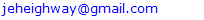Abstract

The intent of this paper is to introduce a new understanding of gravitational phenomena based upon the revolutionary concept of Variable Rest Mass. At the outset, it is postulated that at any particular location, all quantities of like dimensionality are affected by the action of gravity in precisely the same manner and to the same degree. Accordingly, the theory underlying the variability of all rest masses is provided by Niels Bohr’s theory of the hydrogen atom, in the light of which, it is obvious that the gravitational red shift is caused by the gravitational reduction of the electron rest mass. Likewise, the gravitational dilation of measuring rods, a phenomenon still unrecognized by today’s physicists, is also an obvious consequence of general rest mass reduction according to Bohr’s theory for the diameter of the hydrogen atom. Importantly, the concept of rest mass reduction enables a truly satisfying understanding of gravitational potential energy: the change in gravitational potential energy of a body is manifest in the body itself as a change in its rest mass energy. Thus, gravitating masses interact directly, undermining the status of the gravitational field as an energetic field entity. The introduction of a new (Fermat) metric correcting for clock slowing and measuring rod elongation proves that light does not couple to gravity. This metric also reveals the true structure of the black hole. Finally, the formulation of a cosmology that assumes homogeneity but also conserves momentum, is achieved by allowing rest masses to evolve exponentially according to the function presently thought to describe the expansion of the universe.

Keywords: Gravitational rest mass reduction, Gravitational length dilation

Cite this paper: Jack Heighway , Reforming the Theory of Gravity, International Journal of Theoretical and Mathematical Physics, Vol. 10 No. 5, 2020, pp. 91-97. doi: 10.5923/j.ijtmp.20201005.01.

### 1. Introduction

In order to understand how present misunderstandings came about, the presentation follows the history of the development of the present theory of gravity, detailing in particular Einstein’s error in his original but flawed analysis of the gravitational red shift, leading eventually to the universal acceptance of the magical concept of Gravitational Time Dilation, hypothesized ad hoc to permit the pretense of understanding.
Ironically, the root misunderstanding of the gravitational red shift can be traced to its discoverer.

### 2. Einstein

“On the Influence of Gravitation on the Propagation of Light,”
In his wonderfully prescient paper  of 1911, Einstein, invoking his principle of equivalence, predicted the gravitational red shift and offered a simple, convincing argument that the entire reduction of energy occurs at the source. Einstein reasoned that if the frequencies observed at both the source and the receiver were actually correct, the number of wavecrests in the interval between would continually increase, contrary to the assumption of time independence. Since the number of wavecrests in the interval must be constant, whenever a wavecrest enters the interval, one must leave: no change occurs in flight. Einstein thus correctly concluded that the entire red shift must occur at the source. To understand why no frequency reduction is observed at the source, Einstein intuited that clocks, the instruments used to measure frequency, would be slowed in proportion to the frequency reduction, thereby predicting another new phenomenon, gravitational clock slowing.

### 3. Missing a Vital Point

Unfortunately, although Einstein did remark that the frequencies measured with corrected clocks would be the same at the source and the receiver, he did not draw the obvious and correct conclusion that the light moved upward with constant frequency, thus missing the discovery that light does not couple to gravity, a fact still not accepted in the present canon of physics.

### 4. Einstein’s Blunder

Having convinced himself that clocks are slowed in a gravitational field, Einstein sought to understand the fact that observers at the clock would nevertheless measure the speed of light to be unaffected, in accord with the principle of relativity. Seeming to ignore the fact that the measurement of velocity requires the measurement of distance as well as time, Einstein did not consider the possibility that gravity might cause an elongation of measuring rods, an effect that would reduce the distance measured, matching the reduced time observed, thus ensuring that the measured value of the speed of light would equal the true value absent gravity. In fact, this phenomenon, gravitational length dilation has hardly been recognized1 precisely because of Einstein’s unfortunate choice in this issue – adopting, the counter-intuitive idea that the true speed of light is reduced by gravity so that the measured speed of light is unaffected. It was clearly an ad hoc assumption, unsupported by any argument. In effect, Einstein boldly asserted that whatever caused the red shift frequency reduction and the slowing of clock rates, also caused the slowing of the speed of light. Today, Einstein is given credit for the introduction of the concept of gravitational time dilation: the slowing of the flow of time itself, an inexplicable concept presently accepted by the majority of physicists, who also do not realize that the proof that light does not couple to gravity is in the 1911 paper.

### 5. The Road Not Taken

In the century following the publication of the 1911 paper, no one seems to have considered what Einstein might have concluded had he considered the red shift as an increase in wavelength, rather than a decrease in frequency. Einstein’s argument proving that the entire effect occurs at the source would have held unchanged, but the fact that no increase in wavelength would have been observed at the source would have required that the actual size of the device used to measure wavelength, (a diffraction grating or an interferometer), must have increased in every linear dimension in exact proportion to the increase in the wavelength of the light. This effect, gravitational length dilation, might have been predicted by Einstein a century ago, thereby averting the near-universal acceptance of the mind-numbing concept of gravitational time dilation.

### 6. Gravitational Length Dilation: Hidden in Plain Sight

The above argument has remained unthought-of for more than a century. It is natural to ask whether there is any alternative scheme by which gravitational length dilation might have been discovered. In fact, all that is required is a consideration of the two techniques for the measurement of distance. First, there is the direct application of a measuring rod, applied end for end along the course. Electromagnetic echo ranging is the alternative. In the latter technique, the time of flight is measured with a real clock, which is slowed in a gravitational field, resulting in an undervaluation of the distance. Since the two techniques must give the same result, it is clear that measuring rods must be elongated by the action of the gravitational field.
Sticklers will insist on direct proof: For the metric,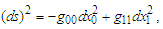The distance by measuring rod is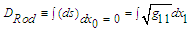For echo ranging, set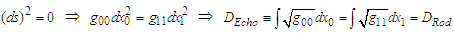### 7. Rehabilitating the Speed of Light

Since the measurement of time and distance are reduced by the same factor, it is clear that the true speed of light and the measured speed of light are equal everywhere in a gravitational field. The scientific community will surely celebrate when this fact is finally recognized.

### 8. The Cause of the Redshift

Einstein made no comment regarding the cause of the red shift – for him it was simply a consequence of the principle of equivalence. To a dedicated experimentalist, the natural focus of an investigation would be on the atom or other quantum system emitting the radiation on the one hand, and, on the other hand, the apparatus used to measure the effect: Emitter and Detector, both ‘clocks’ in a functional sense.
This approach has been rejected by most physicists. Benesh Hoffmann has declared “…the gravitational red shift does not arise from changes in the intrinsic rates of clocks. It arises from what befalls light signals as they traverse space and time in the presence of gravitation.” Better informed physicists also hold that clocks and sources are not physically altered, but reject the in-flight reduction of frequency, accepting, as they do, Einstein’s argument that the entire red shift occurs at the source. They attribute the reduction of the source frequency to gravitational time dilation, according to which, the flow of time itself is reduced by the action of the gravitational field. They also believe that all dynamic phenomena are driven by the flow of time, accounting, not only for the red shift, but for the reduction of the speed of light as well.
Hans Ohanian has given a seemingly cogent argument for accepting time dilation and the rejecting the idea that clocks of themselves are responsible for the red shift: “We have no choice but to accept time dilation as a formal consequence of the theory of gravity in which d𝜏 = (g00)1/2dt for any static field, since, according to the principle of equivalence, it is impossible for the gravitational field to influence the rate of a freely falling clock in any other way.” Additionally, gravitational time dilation is seen as a legitimate phenomenon justifying Einstein’s ad hoc assumption that the true speed of light is reduced in a gravitational field.
But, as will be proved directly, there actually is a phenomenon that can influence the rate of a free-falling clock: gravitational rest mass reduction, arguably the most fundamental of all gravitational phenomena, to date unrecognized by the community of physicists.

### 9. The Dimensionality Postulate

It is an observed fact that on-site measurements of the characteristics (mass, physical size, resonant frequencies, etc.) of real objects and devices are unaffected by gravity, even though remote observations may reveal actual changes of such characteristics. For example, the on-site measured wavelength of the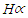line will be 656.281 nm, whether measured in Denver or Death Valley. This is true despite the fact that satellite observations of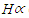emissions coming from these sites would measure red shifts – some for Denver, more for Death Valley. These facts are easily understood: at any particular site, the instruments used to make measurements will be of the same dimensionality as the thing measured, and so will be affected in exactly the same way, rendering real affects undetectable. More generally, it is postulated that at any particular location, all quantities of like dimensionality are affected by the action of gravity in precisely the same manner and to the same degree: for example, the wavelength of light and the spacing of the rulings of a diffraction grating.

### 10. Bohr’s Fundamental Intervals of Time and Distance

The failure of the gravitational physicists to give proper consideration to the work of Niels Bohr, is hard to understand. Bohr’s theory of hydrogen  presented fundamental expressions for the characteristic frequency spectrum and diameter of the hydrogen atom. Thus, Bohr’s expression for the Rydberg,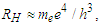clearly shows that the gravitational reduction of rest mass is the true cause of the gravitational red shift. Likewise, Bohr’s formula the for diameter of the hydrogen atom,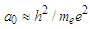indicates that all objects will suffer elongation as a result of rest mass reduction. Clearly, Bohr’s equations identify gravitational rest mass reduction as the most fundamental gravitational effect.

### 11. The True Nature of Gravitational Potential Energy

Ask any modern-day physicist to specify the actual location and physical composition of gravitational potential energy, and you will perhaps be scolded for asking a silly question, but you will never receive an answer.
The concept of rest mass reduction enables a truly satisfying understanding of gravitational potential energy: the change in gravitational potential energy of a body is manifest in the body itself as a change in its rest mass energy: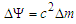. This function, defined in terms of reduced rest mass,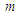, and the proper rest mass,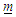, is, to first order in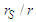, where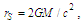equivalent to the usual potential energy,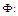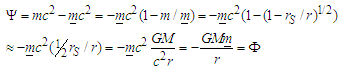This definition puts to rest the difficult concept of a gravitational field possessing a negative energy density, as was thought necessary to account for the attractive nature of gravity.

### 12. Correcting the Metric

Now consider the metrical implications of gravitational clock slowing and gravitational length dilation. The proper metric of a gravitational field satisfying Einstein’s equations does not present an accurate description of the spacetime. Its function, one might say, is to enable the description of the behavior of clocks, rulers, and test bodies that may inhabit the field. A correct description of spacetime requires that corrections be made for the slowing of clock rates and the elongation of measuring rods. Since intervals of time and space are under-valued by the same factor,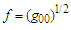, the proper metric in a time-independent field,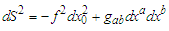, gives rise to a corrected metric, reasonably called the Fermat Metric, by dividing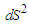by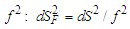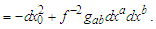The subscript honors Pierre de Fermat (1607–1665) for his introduction of the principle of least time.
Because light signals propagate at constant frequency (as was established in the discussion of the red shift), the flow of true time,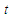, which evolves as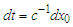, can be propagated to all observers from a single master clock. All stationary observers thus have access to the same metronome, so to speak. Any two such observers will agree regarding their mutual separation by employing electromagnetic echo ranging, with time intervals determined using signals from a master clock. Such distance measurement will permit a master clock to send a start signal to any particular observer, so as to synchronize that observer’s clock with the master clock. Universal Time is thus possible in any static gravitational field.

### 13. No Coupling of Light to Gravity

Fermat was certainly not aware of the existence of the two distinct species of time that figure prominently in Einstein’s theory of gravity. It is clear that the time as measured by local clocks slowed by the factor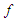is out of consideration for determining the path of a light ray. Thus the principle of least time minimizes the integral of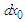over the path of the light ray, for which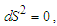implying, for either metric. But regarding spatial geodesics, the two metrics do not give the same paths. For the proper metric,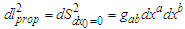, which does not conform to the equation for the ray path. Accepting this metric would suggest that the force of gravity causes a deflection from the geodesic path. But for the Fermat Metric, the spatial geodesic equation,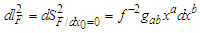, is identical to the equation for least time, indicating that gravity does not couple to light, i.e., to the free electromagnetic field.

### 14. Optical Geometry

There is a compelling argument supporting the assertion that the Fermat Metric correctly describes the geometry of space in a static gravitational field, whereas the Proper Metric does not. Originally, the Fermat name was only attached to the purely spatial metric,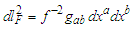. This metric was rediscovered, one might say, in 1988 in the light of a truly profound discovery regarding the kinematics of massive bodies in a gravitational field. Abramowicz et al.  proved that in a static gravitational field, a body will not experience speed-dependent forces (centrifugal and Coriolis forces) if it is constrained to move along a path that a light ray might follow. By analogy to Newtonian mechanics, in which such forces vanish for bodies moving in straight lines, the authors defined a new geometry by identifying light ray paths as the geodesics of the new geometry, which they named, appropriately, ‘Optical Geometry’. The metric of Optical Geometry is identical to the geometric part of the complete Fermat Metric, which has been introduced in this paper to correct for slowing clock rates as well as the elongation of measuring rods.

### 15. The ‘Bending of Light’ – the Consensus View

Generally, textbooks present the calculation for the deflection according to Einstein’s theory, tacitly accepting that any deflection establishes the coupling of light to gravity, the only question being whether eclipse measurements in the field agree with the theory. There is no discussion as to whether the trajectory of a light ray deviates from a pure spatial geodesic, as would be required to prove that light feels the force of gravity. The argument presented above for non-coupling is simple but subtle compared to the stark simplicity of the argument in the case of the simple gravitational red shift.

### 16. Neither Light nor Gravity Acts as a Source of Gravity

The fact that gravity does not couple to light implies that the free electromagnetic field does not contribute to the gravitational field, upsetting Einstein’s assumption that every form of energy will act as a source of the gravitational field. This is highly significant in itself, but perhaps even more telling are the implications for any theory of quantum gravity that may be proposed. Since gravity is a long-range field, the graviton would be massless and so, like the photon, would follow a Fermat geodesic path, proving that gravitons would not couple with the gravitational field, nor act as sources for the gravitational field.

### 17. An Important Caveat Regarding Massless Fields

It is vitally important to understand that only free massless fields, e.g., photons and gravitons in flight, do not couple to gravity. In contrast, the kinetic energy of a massless field that is confined does possess an effective rest mass, is affected by gravity, and does contribute to the gravitational field. Notably, this includes the intense electromagnetic radiation trapped in the interior of a star.
As a very crude gedankenexperiment, one may imagine raising a box with perfectly reflecting walls containing light of some definite wavelength when the box is at rest. As the box is raised, the wavelength of the light will be unaffected in flight between the walls, but will be reduced on contact with any wall, all of which will be moving inwardly due to the gravitational length dilation effect, thus increasing the energy in the box.
Stunningly, according to Quantum Chromodynamics, 98.8% of the mass of a nucleon exists in the form of the kinetic energy of confined massless gluons which bind the (barely) massive quarks together.
Regarding a static gravitational field a serious question arises: it is easily shown that, in any such field, a reference system (free fall!) can be adopted in which every component of the metric is reduced to Galilean form, implying that any formulation of the energy-momentum tensor vanishes not just in that special frame, but, by the very nature of tensors, in every reference frame. This should be (but hasn’t been) enough to convince everyone that the Fermat metric tensor of a static gravitational field only describes a three-dimensional geometry, rather than an energetic field. The gravitational force between masses may perhaps be mediated by the direct exchange of gravitons emanating from the masses themselves, just as the electric force between charges is mediated by the exchange of photons. Admittedly, how the presence of a massive object alters the geometry of space is yet to be understood.

### 18. The Non-Spinning Graviton

Considering the fact that the metric of a static gravitational field is related by a conformal transformation to the Fermat metric, which describes mere geometry uncoupled to a universal Newtonian time, attempts to quantize the gravitational field would seem to be a lost cause. But for those determined to pursue the aim of developing a viable theory of quantum gravity, there is a welcomed surprise: the presumed graviton cannot be a spin-two boson – it would have to be spinless. Theorists have rejected the scalar spin-zero graviton on the basis of the observational fact that light rays appear to be ‘bent’ by gravity. The rejection follows from the fact that the spin-zero graviton can only couple to the trace of the energy-momentum tensor of a target field, and that tensor for the free electromagnetic field has a zero trace. But, as we have seen, light rays actually do follow the spatial geodesics of the correct Fermat Geometry, indicating that there is no coupling of light to gravity. Thus, the spin-zero graviton would have to be accepted since it cannot couple to the free electromagnetic field, while the spin-two graviton would have to be rejected because it would couple to the complete energy-momentum tensor of the electromagnetic field.
The fact that gravitons would interact neither with the gravitational field itself, nor with one another, would eliminate dreaded non-linearities, and may give promise of a linear, spin-zero scalar theory – a radical and welcomed simplification of the problem of formulating a quantum theory of gravity.

### 19. The True Nature of a Black Hole

Current opinion holds that the basic structure of a black hole consists of a singularity hidden behind a surface called the event horizon, from which the escape velocity is equal to the speed of light. This surface is very peculiar in that light sent from a finite proper distance directly toward the event horizon never reaches that surface! Proponents of the accepted interpretation ‘explain’ that because of ‘the slowing of the flow of time itself’, the speed of light goes to zero as light approaches the event horizon.
The puzzling phenomena that appear in the conventional interpretation are easily understood in terms of the Fermat metric for the Schwarzschild field. According to this metric, the area of a centered sphere is not a monotone function of the radial coordinate, r: it has a minimum at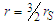, the locus of photon orbits. This sphere of minimum area, the stenosphere, is the throat of a wormhole-like structure that magically connects our familiar universe to a separate infinite three-dimensional space. Nothing, not even light, can reach the event horizon, since that ‘surface’ is just the infinity of ‘innerspace’. The controversy regarding the supposed loss of information and entropy when matter ‘disappears’ behind the event horizon is settled: nothing disappears: nothing is lost. Finally, the baleful singularity, which is supposed to lurk behind the event horizon, is banished to the non-existent region beyond innerspace infinity.

### 20. Noether’s Cosmology

The most surprising implication of the variable rest mass concept relates to cosmology. Hubble’s 1929 observational discovery of the systematic distance – redshift relationship appeared to confirm the mathematical models of Friedman, and independently,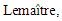predicting the expansion of the universe. Immediately, it was widely accepted that galaxies are actually flying through space away from us and from one another – the further, the faster. This in turn suggested that the universe grew from an incredibly hot and dense condition (a singularity!) billions of years ago. Later, theorists explained that it should be understood that the galaxies are not actually moving through space, but rather that space itself is expanding. Thus, the conventional understanding of the cosmological red shift holds that the wavelength of light is continually stretched in flight by the expansion of space.
Neither astronomers nor cosmologists seem to be concerned with the fact that this explanation of the cosmological red shift fails to conserve the momentum of the observed radiation, as Noether's Theorem  demands in a spatially homogeneous universe. Observation shows that, on a sufficiently large scale, the universe is homogeneous and isotropic to a very high degree, and virtually every cosmological model assumes homogeneity at the outset. Perhaps no one has even considered this problem since, as every observation of the red shift appears to have demonstrated, momentum seems manifestly to be not conserved. The cosmic microwave background radiation that we detect today has a thermal black body spectrum at 3°K: (peak wavelength one millimeter). These photons were born in a 3000°K hydrogen-helium plasma (peak wavelength one micron) at ‘recombination’ time, when the plasma first became transparent. Their wavelength has seemingly increased by a factor of about 1000 – how is it possible that their momentum has not changed?
The only possibility is that over the aeons, measuring instruments have changed, and are changing, decreasing their characteristic wavelengths – for example, diffraction gratings have shrunk and are shrinking. Likewise, atomic and molecular frequencies are continually increasing, as are clock rates. It turns out that the only way this might occur is if the rest masses of all massive bodies everywhere have been, and are, increasing in proportion to a(t) = A(t), in which A(t) is the function that is presently interpreted as representing the increasing scale of the universe, and Ã is its present value. One may facetiously observe that the universe is not expanding – it’s just that everything in it is shrinking.
To prove that momentum conservation requires rest masses to increase in proportion to a(t), one need only consider the motion of a test mass through space. In this case, there is an integral of motion  for the Friedman metric, namely, a(t)∙β(1β2)−½ = constant. But since momentum is mc β (1–β2)−½, it is clear that conservation of momentum requires that the true rest mass, m, must be proportional to a(t), that is,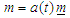where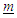is the measured rest mass, a constant.
The solution of Einstein’s field equations for cosmology under the assumption that rest masses increase in proportion to a(t) (to ensure momentum conservation) is presented in reference . The mass evolution function, a(t), turns out to be a simple exponential function of world time: a(t) = exp (–H0 (tnow t)), where H0 is the Hubble constant.
Regarding the evolution of proper time,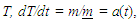so,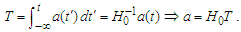Thus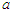is a linear function of proper time, T. Paradoxically, it is clear that the universe is infinitely old in terms of world time, t : yet in terms of proper time, T, it may be said that the universe suddenly came into existence with a ‘Big Bang’ some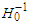seconds ago (~14 billion years ago).
One could say that proper time is simply what atomic clocks indicate, but world time requires special consideration. It seems appropriate to define the two types of clocks in an operational way, one keeping proper time, the other, world time. Each has the form of a ‘light clock’ incorporating an emitter of light pulses and a remotely located mirror which reflects the pulses back to the source where they are counted, driving the clock. For the clock keeping proper time, the source and reflector are connected by a ‘rigid’ framework (which, in fact, shrinks as rest masses increase). The clock keeping world time employs two unconnected satellites which keep a constant true separation by using active thrusting, if necessary, in order to maintain an unchanging standing wave, comprising a fixed number of nodes, in the space between them.
The phenomenon of universal rest mass evolution appears to have been forced upon us by the demand of momentum conservation. Immediately the question arises as to what physical mechanism may justify this seemingly ad hoc, ‘save the phenomenon’ assumption. One promising mechanism depends upon the existence of the Higgs Field and its associated quantum particle, the Higgs Boson. The Higgs boson transfers energy, and hence mass, from the massive Higgs Field to all other fundamental massive particles, including not only electrons, protons and neutrons, but also to the Higgs itself. If, at any given time, the intensity of the Higgs Field increases with time in proportion to its present value, the exponential growth pattern demanded to assure conservation of momentum is satisfied.

### 21. Summary

In this paper several important concepts, very far out of the mainstream, have been introduced. I hope that readers have been able to set aside their allegiance to currently accepted theory long enough to follow the logic of the arguments presented. These draw conclusions that in several instances go counter to certain long held beliefs that are, in fact, simply unwarranted assumptions. Among these one may list:
1. The measurement of distance by measuring rod is unaffected by gravity
2. Gravitational Time Dilation is a real phenomenon. It slows clock rates, and is the cause of the gravitational red shift and the reduction of the speed of light
3. Rest mass is not affected by gravity
4. The gravitational potential energy of a body resides in the gravitational field
5. All forms of energy are affected by gravity and act as sources of the gravitational field
6. The proper metric represents the true structure of spacetime
Recently developed (incorrect) concepts backed by tentative theory include:
7. Everything entering a black hole vanishes behind the event horizon, (the ‘No Hair’ theorem)
8. The graviton is a spin-two boson
9. The cosmic red shift results from the expansion of space itself, stretching wavelengths in flight
10. The universe is homogeneous, but photon momentum is not conserved, contra Noether
11. The universe created itself out of nothing in an everywhere-at-once event, the Big Bang
On the other side of the ledger, one may list the new ideas introduced in this paper, to which the mainstream community of physicists may object:
1. Gravitational Rest Mass Reduction and Gravitational Length Dilation are real phenomena
2. Changes in the gravitational potential energy of a body exist as changes in its rest mass energy
3. The introduction of the Fermat Metric, correcting for slow clocks and elongated measuring rods, has led to the following six important conclusions:
4. Light does not couple to gravity
5. The graviton, if presumed to exist, must be a spin-zero boson
6. As a massless free field, gravity does not couple to itself: nor does it act as source of the gravitat 84
7. The ‘No-Hair’ theorem for black holes is false
8. There is no black hole singularity
9. Black holes connect our universe to innerspace, a separate, infinite, three-dimensional space. (This is admittedly a finding not wished for!)
10. Cosmic homogeneity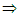momentum conservation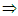rest masses increase as A(t)the frequencies of measuring devices increase as A(t)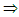the observed cosmic redshift
11. Finally, in terms of world time, the universe has always existed: there was no Big Bang

### Note

1. R H Dicke  recounts the findings of a group of theorists, including Rosen, Gupta, Feynman and Thirring, who proved that the gravitational field can be treated as an ordinary zero-mass, chargeless tensor field, and that its interaction with matter causes the dilation of meter sticks and the slowing of clock rates. These effects were shown to admit a simple correction: the metric is simply multiplied by a single coordinate-dependent scale factor redefining the units of length, time and reciprocal mass. Clearly, the fact that the scale factor includes the reciprocal mass, indicates that the gravitational field causes a reduction of rest mass in addition to the dilation of meter sticks and the slowing of clock rates. Unaccountably, this was not noted in the Dicke’s account. In fact, as will be proved, rest mass reduction is actually the cause of the dilation of meter sticks and the slowing of clock rates!

### References

  Einstein, A., “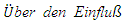der Schwerkraft auf die Ausbreitung des Lichtes”, Annalen der Physik, 35, (1911) 898– 908. English translation: “On the Influence of Gravitation on the Propagation of Light,” in The Principle of Relativity, Dover Publications, Inc. (1952) 99–108.  Dicke, R. H., “The Theoretical Significance of Experimental Relativity” Gordon and Breach Science Publishers, Inc. (1965) 14-15.  Bohr, Niels (1913) "On the Constitution of Atoms and Molecules, Part I" (PDF). Philosophical Magazine. 26 (151): 1–24. doi: 10.1080/14786441308634955.  Abramowicz, M. A., Carter B. and Lasota J-P., “Optical reference geometry for stationary and static dynamics.” Gen. Rel. Grav, 20, (1988) 1173–1183,. http://dx.doi.org/10.1007/BF00758937.  Noether, Emmy (1918), "Invariante Variationsprobleme" [Invariant Variation Problems], Nachr. D. önig Gesellsch. D. Wiss. (in German), Göttingen: Math-phys. Klasse, 1918: 235–257. English translation by M. A. Tavel (1918), arXiv: physics/0503066. DOI: 10.1080/00411457108231446 Explicating briefly: “If a physical process exhibits the same outcomes regardless of place, or time, then linear momentum, or energy, respectively, is conserved.”  Heighway, J., “Einstein’s Theory of Gravity: Misunderstood for a Century”, International Journal of Theoretical and Mathematical Physics, Vol. 7 No. 3, 2017, pp. 41-50. doi: 10.5923/j.ijtmp.20170703.01. Appendix B, 47.  Ibid, Appendix C, 47-49 See also Heighway, J., Einstein, the Aether & Variable Rest Mass, ISBN 978-1-61658-620-1 69-77.